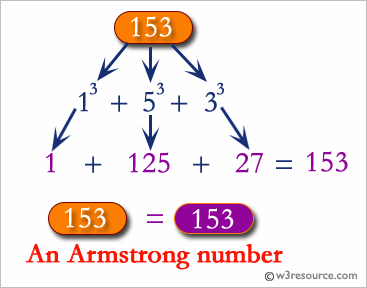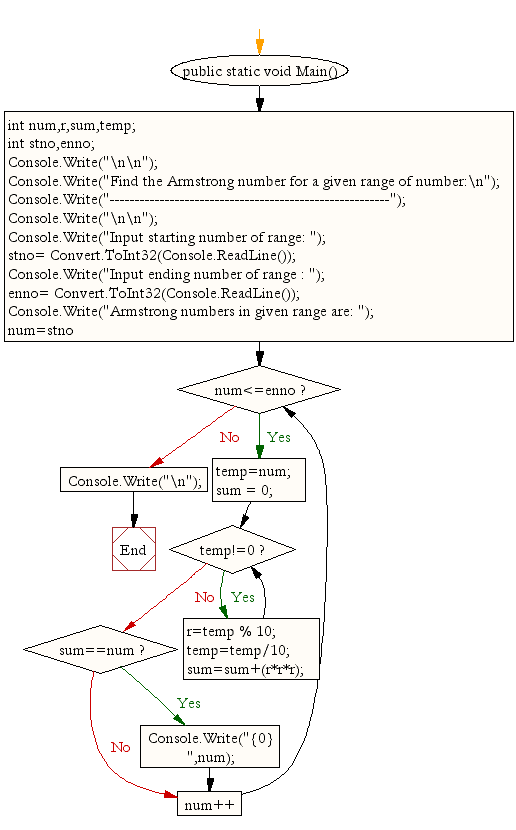﻿ C# - Find the Armstrong number for a given range of number# C# Sharp Exercises: Find the Armstrong number for a given range of number

## C# Sharp For Loop: Exercise-30 with Solution

Write a C# Sharp Program to find the Armstrong number for a given range of number.Sample Solution:-

C# Sharp Code:

``````/*When the sum of the cube of the individual digits of a number*/
/*is equal to that number, the number is called Armstrong number. For example 153.*/
/*Sum of its divisor is 13 + 53;+ 33; = 1+125+27 = 153*/
using System;
public class Exercise30
{
public static void Main()
{
int num,r,sum,temp;
int stno,enno;

Console.Write("\n\n");
Console.Write("Find the Armstrong number for a given range of number:\n");
Console.Write("--------------------------------------------------------");
Console.Write("\n\n");

Console.Write("Input starting number of range: ");

Console.Write("Input ending number of range : ");

Console.Write("Armstrong numbers in given range are: ");
for(num=stno;num<=enno;num++){
temp=num;
sum = 0;

while(temp!=0){
r=temp % 10;
temp=temp/10;
sum=sum+(r*r*r);
}
if(sum==num)
Console.Write("{0} ",num);
}
Console.Write("\n");
}
}
```
```

Sample Output:

```Find the Armstrong number for a given range of number:
--------------------------------------------------------
Input starting number of range: 1
Input ending number of range : 500
Armstrong numbers in given range are: 1 153 370 371 407
```

Flowchart:C# Sharp Code Editor:

Contribute your code and comments through Disqus.

What is the difficulty level of this exercise?

Test your Programming skills with w3resource's quiz.

﻿